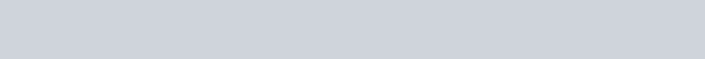# Gradient Descent Optimization using TensorFlow in Python

Hey Everyone,

In this article, we will be understanding one of the Most Complex terminologies of Machine Learning which is Gradient Descent.
I will try my level best to explain this terminology as simple as possible.
Before we get started with the Gradient Descent Optimization using TensorFlow in Python we will be understanding two terminologies of Machine Learning.

## Let’s understand the terms with an exampleI am a professor of a subject at a University. I provide my two favorite students name Arun and Kamlesh question bank for a Term Test Examination.
During the term test, I hand them question papers which is a combination of questions from the question bank and outside question bank.
While checking their paper I realized that Arun had has not answered the questions appropriately which were asked from the question bank.
Kamlesh, on the other hand, answered the questions appropriately but failed to appropriately answer the question asked outside question bank.

The performance of Arun explains the Underfitting of the model while the performance of Kamlesh explains the Overfitting of the model in Machine Learning.

When the model does not do well on seen data while training the model, This phenomenon is called, “Underfitting“.
While when the model does well on the seen data but doesn’t perform well on the unseen data, This phenomenon is called, “Overfitting“.

Hence when a model does well on the seen data doesn’t necessarily mean that the Model is Actionable.

To Prevent Underfitting/Overfitting of the Model Gradient Descent Optimization plays a crucial role.
The loss function of the model tells us how good the model is in making predictions.

## Loss Function formula:Let’s break this term into two parts Gradient meaning “slopes which can be upward or downward” and Descent means to “move downwards”. Now the Goal of the Gradient Descent is to have low local minimal of a differentiable function.

Gradient Descent is an iterative optimization algorithm used to minimize some function by moving towards the steepest descent. We update the parameters of the Model. In the following example, we will be optimizing a linear model. To optimize the parameter we will be manipulating the learning rate of the `GradientDescentOptimizer()`.
There are no set rules to choose a particular learning rate. But if the learning rate is high we might surpass the lowest local minimal whereas the lower learning rate might be time-consuming.
In this tutorial, the Learning rate will be 0.01.## Modules Required:

• NumPy
• Matplotlib
• TensorFlow 2.0
• Sklearn

## Let’s Hop into the Code:

We will be importing all the required modules.

```import tensorflow as tf
import numpy as np
import matplotlib.pyplot as plt
from sklearn.utils import shuffle

print("All required Modules are Imported")```

Output:

`All required Modules are Imported`

Now let us load the Boston Housing Price dataset

```"""
Load and return the boston house-prices dataset

"""

Output:

`Dataset has been Loaded`

A good characteristic of a Machine learning Engineer is that they Shuffle the data in the dataset. So that we don’t train the model on summer data and expect to perform on winter data. Also, we will be Scaling the Train and Test data.

```X, Y = shuffle(X, Y)

X_train = (X[:300]- X[:300].mean())/X[:300].std()
X_test = (X[300:] - X[300:].mean())/X[300:].std()

Y_train = (Y[:300] - Y[:300].mean())/Y[:300].std()
Y_test = (Y[300:] - Y[300:].mean())/Y[300:].std()
print("Shuffling and Scaling has been done")```

Output:

`Shuffling and Scaling has been done`

We will be Mentioning TensorFlow variables, `weights` which will be having random numbers normally distributed & `intercepts` for Developing the Linear Equation mentioned belowWe will be coding the above equation, square the loss while predicting, taking the mean of the square of loss.

```pred = tf.add(intercepts, tf.matmul(x, weights))

squared_deltas = tf.square(y - pred)

loss = tf.reduce_mean(squared_deltas)

init = tf.compat.v1.global_variables_initializer()

cost_history = []```

The Learning rate for Gradient Descent is 0.01.

Appending only selective set of loss into the `cost_history` and visualizing the loss in every 10 Epochs of 5000 Epochs.

```"""Initializes the  the Global Variable Initializer, Append loss after every 10 epochs, print the loss after every 500 epochs and Plot the cost_history"""
epochs = 5000

print("The Loss after every 500 epoch are: ")
print("--------------------------------------------------------")
with tf.compat.v1.Session() as sess:
sess.run(init)

for i in range(epochs):
sess.run(optimizer, {x: X_train, y: Y_train})

if i%10 == 0:
cost_history.append(sess.run(loss,{x: X_train, y: Y_train}))

if i%500 == 0:
print(f"Loss at {i}th Epoch:")
print(sess.run(loss,{x: X_train, y: Y_train}))

plt.plot(cost_history)
plt.show()
print("Error on the Test Data: ",sess.run(loss, {x: X_test, y: Y_test}))

sess.close()```

Output:We have now fairly understood the importance of Gradient Descent and its Learning rate for optimization of the model by lowering the loss/cost function.

I hope you had a great time learning.
I highly recommend my readers to manipulate the Epoch value and look at its output.
This topic initially might look very complex and hard to understand. I would highly appreciate my readers if they go through this article again and again.
Just for your information, we have an optimization technique that is more sophisticated than Gradient Descent called, “Stochastic Gradient Descent“.
Which we might be covering some other day.